```library(readr)
mort[1:2, 1:5]```
```# A tibble: 2 x 5
X1          `1760` `1761` `1762` `1763`
<chr>        <dbl>  <dbl>  <dbl>  <dbl>
1 Afghanistan     NA     NA     NA     NA
2 Albania         NA     NA     NA     NA```

## Read in Data: `jhur`

`jhur::read_mortality()`
```# A tibble: 197 x 255
X1    `1760` `1761` `1762` `1763` `1764` `1765` `1766` `1767` `1768` `1769`
<chr>  <dbl>  <dbl>  <dbl>  <dbl>  <dbl>  <dbl>  <dbl>  <dbl>  <dbl>  <dbl>
1 Afgh~     NA     NA     NA     NA     NA     NA     NA     NA     NA     NA
2 Alba~     NA     NA     NA     NA     NA     NA     NA     NA     NA     NA
3 Alge~     NA     NA     NA     NA     NA     NA     NA     NA     NA     NA
4 Ango~     NA     NA     NA     NA     NA     NA     NA     NA     NA     NA
5 Arge~     NA     NA     NA     NA     NA     NA     NA     NA     NA     NA
6 Arme~     NA     NA     NA     NA     NA     NA     NA     NA     NA     NA
7 Aruba     NA     NA     NA     NA     NA     NA     NA     NA     NA     NA
8 Aust~     NA     NA     NA     NA     NA     NA     NA     NA     NA     NA
9 Aust~     NA     NA     NA     NA     NA     NA     NA     NA     NA     NA
10 Azer~     NA     NA     NA     NA     NA     NA     NA     NA     NA     NA
# ... with 187 more rows, and 244 more variables: `1770` <dbl>, `1771` <dbl>,
#   `1772` <dbl>, `1773` <dbl>, `1774` <dbl>, `1775` <dbl>, `1776` <dbl>,
#   `1777` <dbl>, `1778` <dbl>, `1779` <dbl>, `1780` <dbl>, `1781` <dbl>,
#   `1782` <dbl>, `1783` <dbl>, `1784` <dbl>, `1785` <dbl>, `1786` <dbl>,
#   `1787` <dbl>, `1788` <dbl>, `1789` <dbl>, `1790` <dbl>, `1791` <dbl>,
#   `1792` <dbl>, `1793` <dbl>, `1794` <dbl>, `1795` <dbl>, `1796` <dbl>,
#   `1797` <dbl>, `1798` <dbl>, `1799` <dbl>, `1800` <dbl>, `1801` <dbl>,
#   `1802` <dbl>, `1803` <dbl>, `1804` <dbl>, `1805` <dbl>, `1806` <dbl>,
#   `1807` <dbl>, `1808` <dbl>, `1809` <dbl>, `1810` <dbl>, `1811` <dbl>,
#   `1812` <dbl>, `1813` <dbl>, `1814` <dbl>, `1815` <dbl>, `1816` <dbl>,
#   `1817` <dbl>, `1818` <dbl>, `1819` <dbl>, `1820` <dbl>, `1821` <dbl>,
#   `1822` <dbl>, `1823` <dbl>, `1824` <dbl>, `1825` <dbl>, `1826` <dbl>,
#   `1827` <dbl>, `1828` <dbl>, `1829` <dbl>, `1830` <dbl>, `1831` <dbl>,
#   `1832` <dbl>, `1833` <dbl>, `1834` <dbl>, `1835` <dbl>, `1836` <dbl>,
#   `1837` <dbl>, `1838` <dbl>, `1839` <dbl>, `1840` <dbl>, `1841` <dbl>,
#   `1842` <dbl>, `1843` <dbl>, `1844` <dbl>, `1845` <dbl>, `1846` <dbl>,
#   `1847` <dbl>, `1848` <dbl>, `1849` <dbl>, `1850` <dbl>, `1851` <dbl>,
#   `1852` <dbl>, `1853` <dbl>, `1854` <dbl>, `1855` <dbl>, `1856` <dbl>,
#   `1857` <dbl>, `1858` <dbl>, `1859` <dbl>, `1860` <dbl>, `1861` <dbl>,
#   `1862` <dbl>, `1863` <dbl>, `1864` <dbl>, `1865` <dbl>, `1866` <dbl>,
#   `1867` <dbl>, `1868` <dbl>, `1869` <dbl>, ...```
```mort = mort %>% rename(country = X1)
mort[1:2, 1:5]```
```# A tibble: 2 x 5
country     `1760` `1761` `1762` `1763`
<chr>        <dbl>  <dbl>  <dbl>  <dbl>
1 Afghanistan     NA     NA     NA     NA
2 Albania         NA     NA     NA     NA```

## ggplot2

Let’s try this out on the childhood mortality data used above. However, let’s do some manipulation first, by using `gather` on the data to convert to long.

```library(tidyverse)
long = mort
long = long %>% gather(year, morts, -country)
```# A tibble: 2 x 3
country     year  morts
<chr>       <chr> <dbl>
1 Afghanistan 1760     NA
2 Albania     1760     NA```

## ggplot2

Let’s also make the year numeric, as we did above in the stand-alone `year` variable.

```library(stringr)
library(dplyr)
long\$year = long\$year %>% str_replace("^X", "") %>% as.numeric
long = long %>% filter(!is.na(morts))```

## Plot the long data

```swede_long = long %>% filter(country == "Sweden")
qplot(x = year, y = morts, data = swede_long)```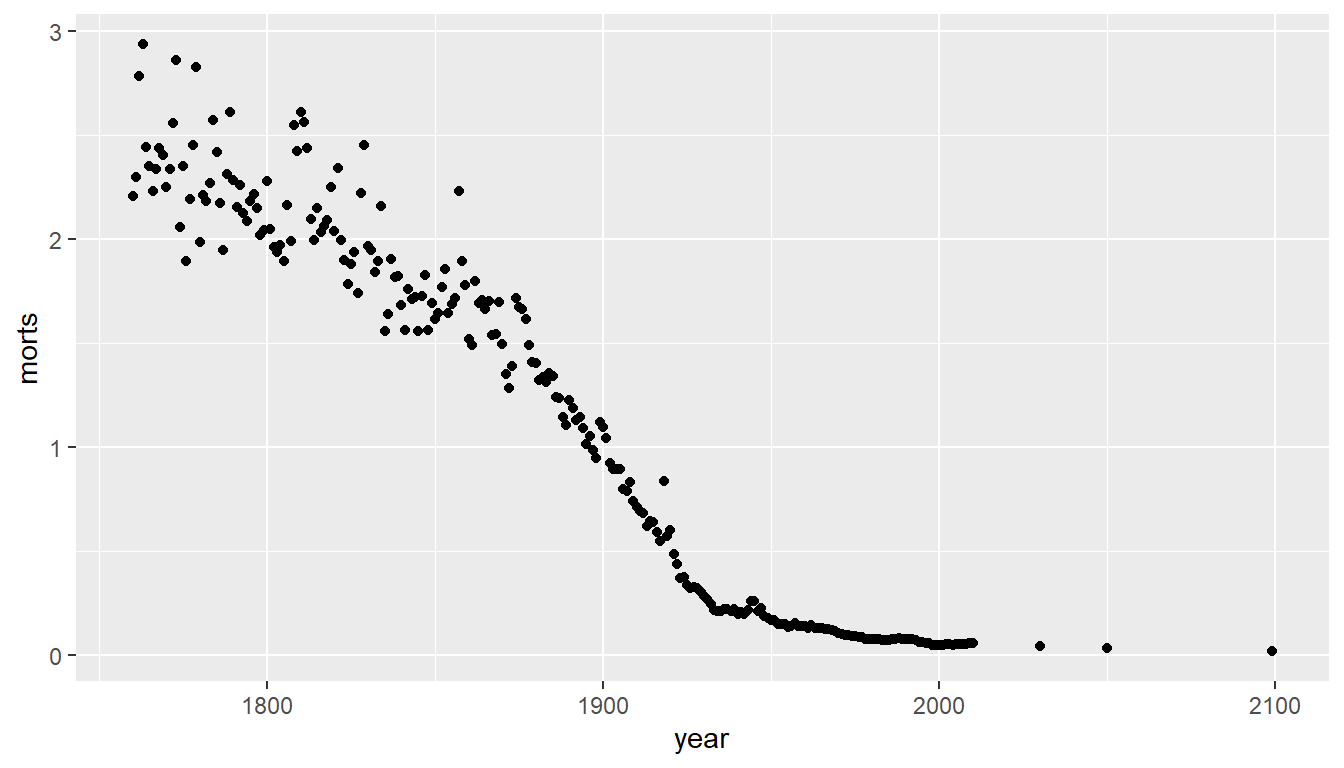## Plot the long data only up to 2012

`qplot(x = year, y = morts, data = swede_long, xlim = c(1760,2012))`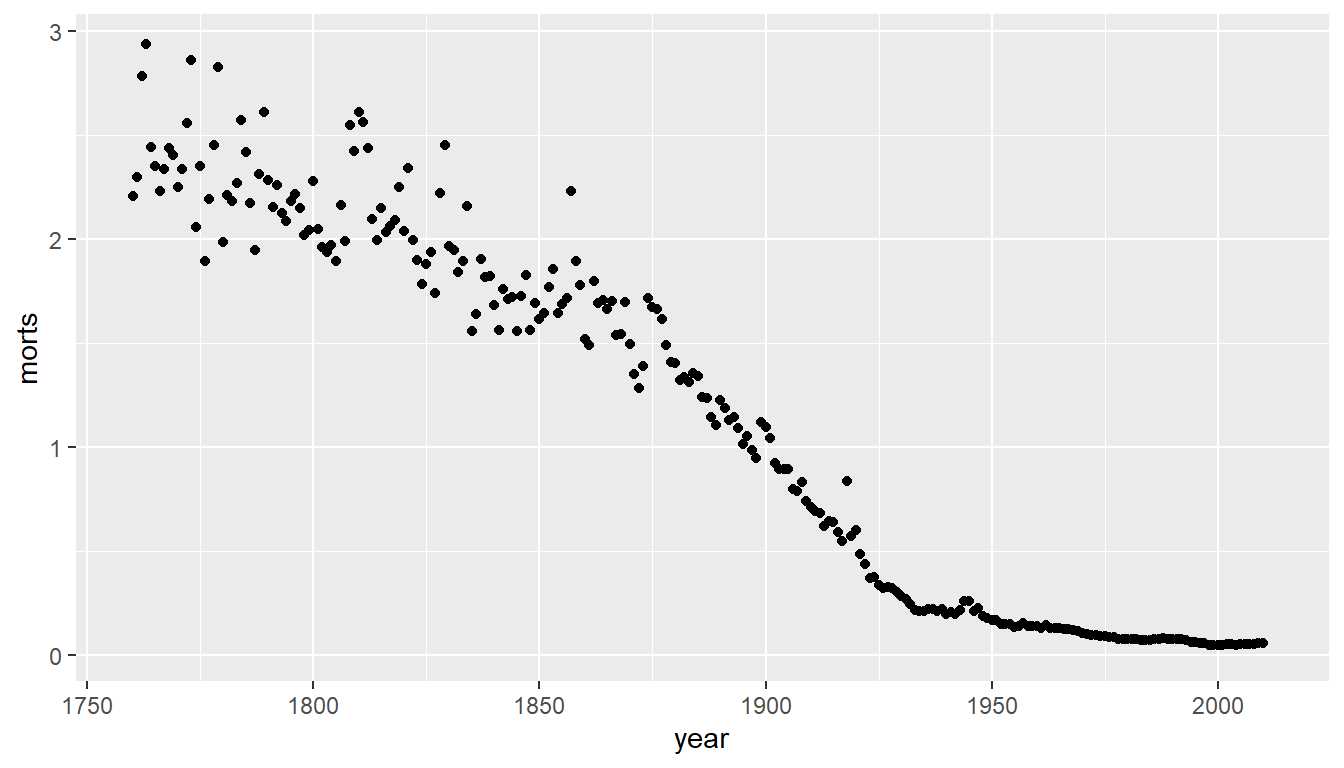## ggplot2

`ggplot2` is a package of plotting that is very popular and powerful (using the grammar of graphics). `qplot` (“quick plot”), similar to `plot`

```library(ggplot2)
qplot(x = year, y = morts, data = swede_long)```## ggplot2

The generic plotting function is `ggplot`, which uses aesthetics:

`ggplot(data, aes(args))`
`g = ggplot(data = swede_long, aes(x = year, y = morts))`

`g` is an object, which you can adapt into multiple plots!

## ggplot2

Common aesthetics:

• x
• y
• colour/color
• size
• fill
• shape

If you set these in `aes`, you set them to a variable. If you want to set them for all values, set them in a `geom`.

## ggplot2

You can do this most of the time using `qplot`, but `qplot` will assume a scatterplot if `x` and `y` are specified and histogram if `x` is specified:

```q = qplot(data = swede_long, x = year, y = morts)
q````g` is an object, which you can adapt into multiple plots!

## ggplot2: what’s a `geom`?

`g` on it’s own can’t be plotted, we have to add `layers`, usually with `geom_` commands:

• `geom_point` - add points
• `geom_line` - add lines
• `geom_density` - add a density plot
• `geom_histogram` - add a histogram
• `geom_smooth` - add a smoother
• `geom_boxplot` - add a boxplots
• `geom_bar` - bar charts
• `geom_tile` - rectangles/heatmaps

## ggplot2: adding a geom and assigning

You “add” things to a plot with a `+` sign (not pipe!). If you assign a plot to an object, you must call `print` to print it.

`gpoints = g + geom_point(); print(gpoints) # one line for slides`Otherwise it prints by default - this time it’s a line

`g + geom_line()`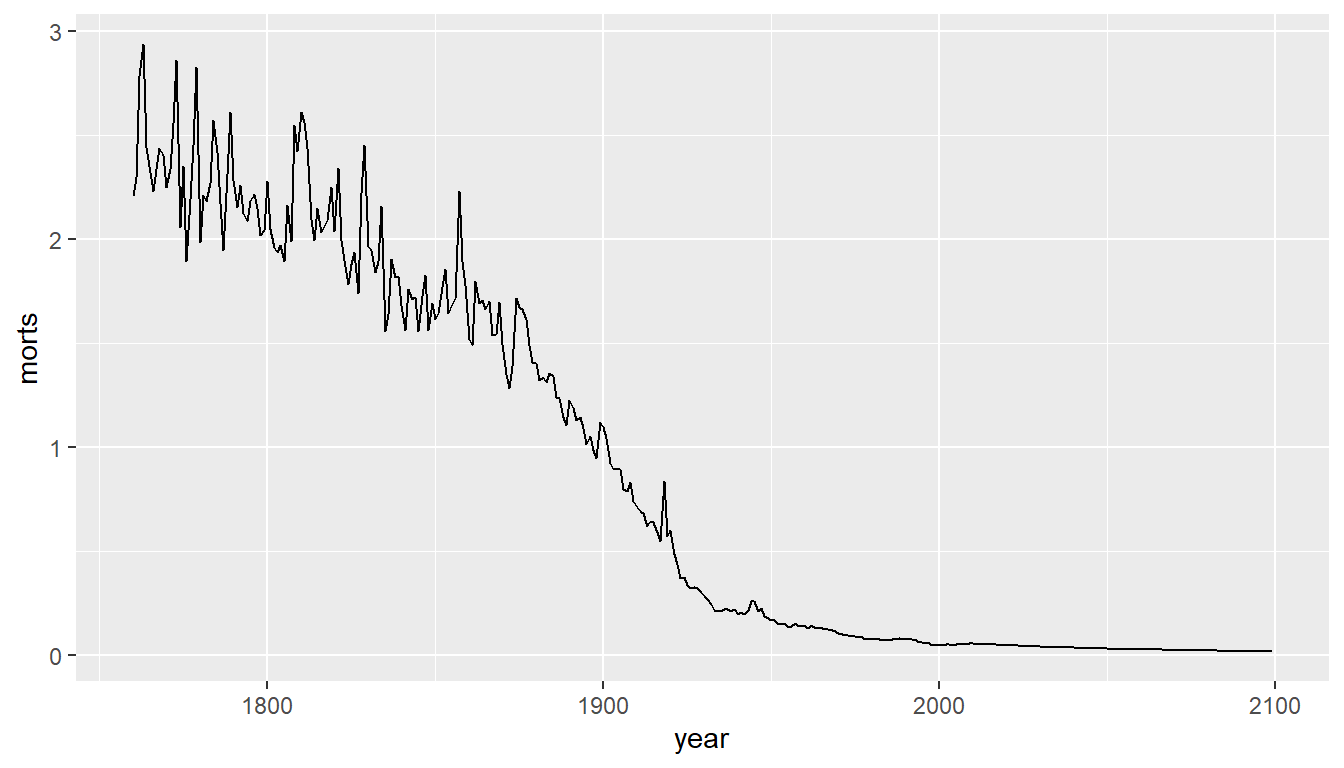`g + geom_line() + geom_point()`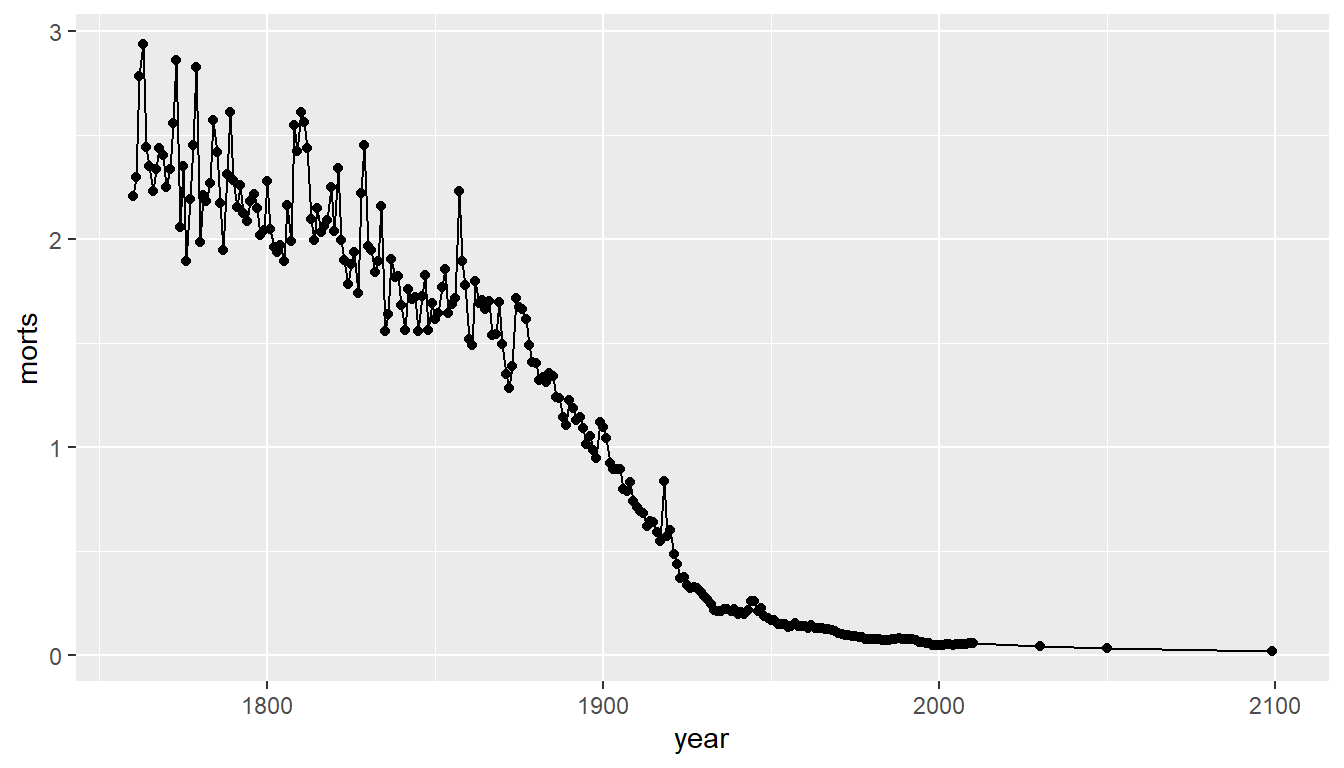Let’s add a smoother through the points:

`g + geom_line() + geom_smooth()`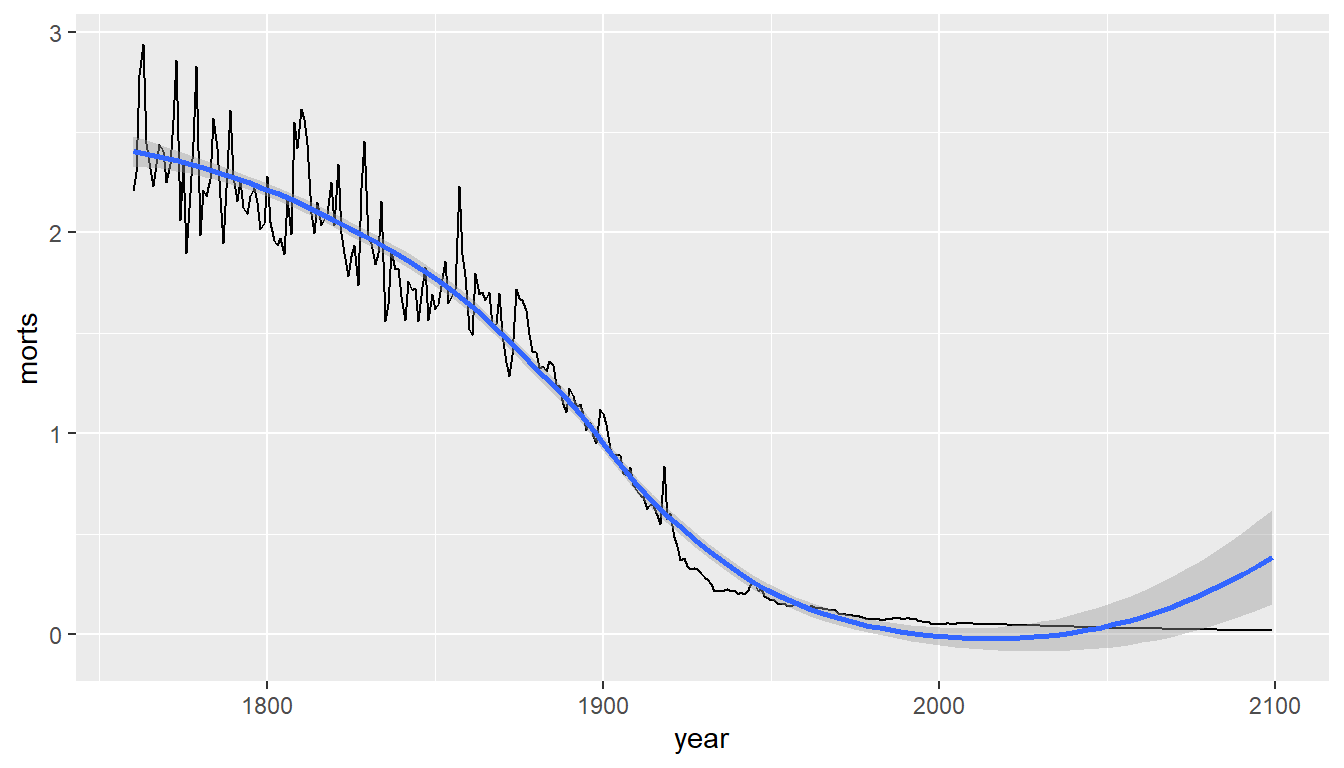## ggplot2: grouping - using colour

If we want a plot with new data, call `ggplot` again. Group plots by `country` using `colour` (piping in the data):

```sub = long %>% filter(country %in% c("United States", "United Kingdom",
"Sweden", "Afghanistan", "Rwanda"))
g = sub %>% ggplot(aes(x = year, y = morts, colour = country))
g + geom_line()```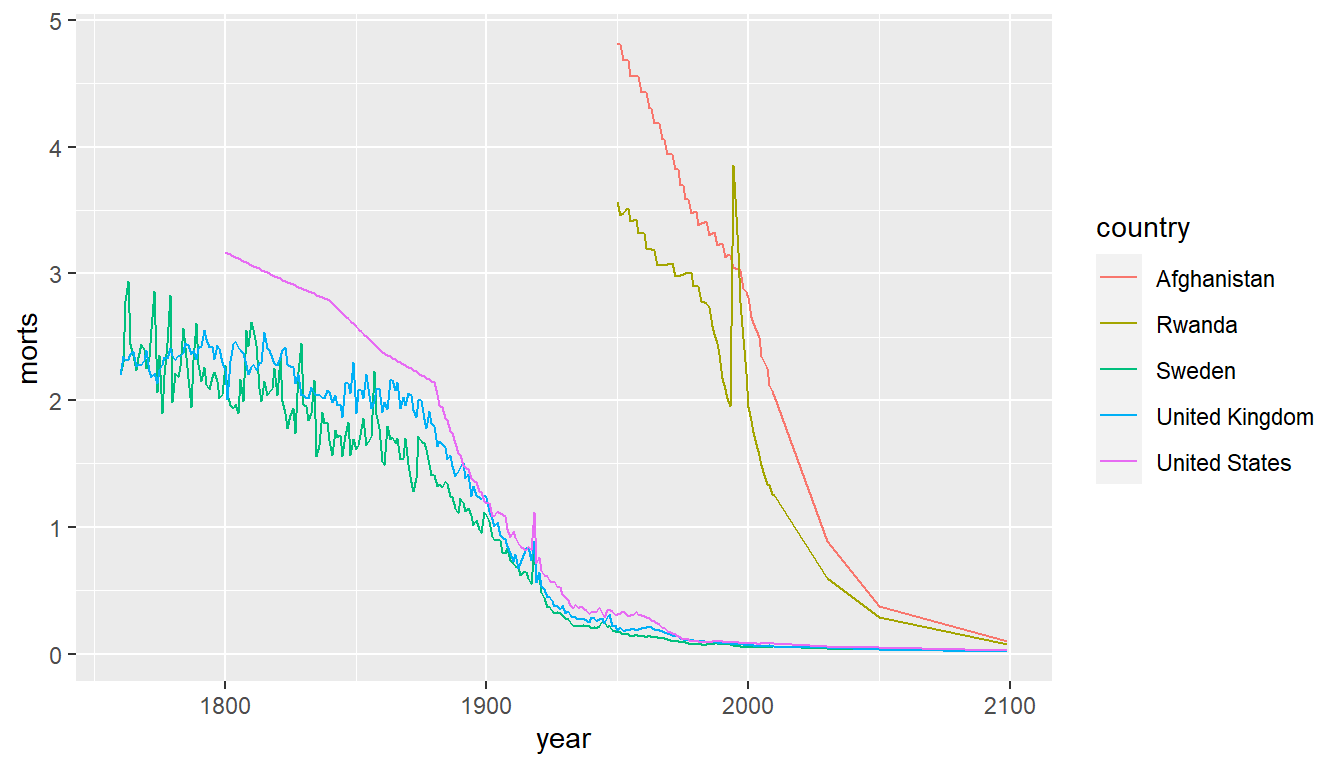## Coloring manually

There are many `scale_AESTHETICS_*` functions and `scale_AESTHETICS_manual` allows to directly specify the colors:

```g + geom_line() + scale_colour_manual(values =
c("United States" = "blue", "United Kingdom" = "green",
"Sweden" = "black", "Afghanistan" = "red", "Rwanda" = "orange"))```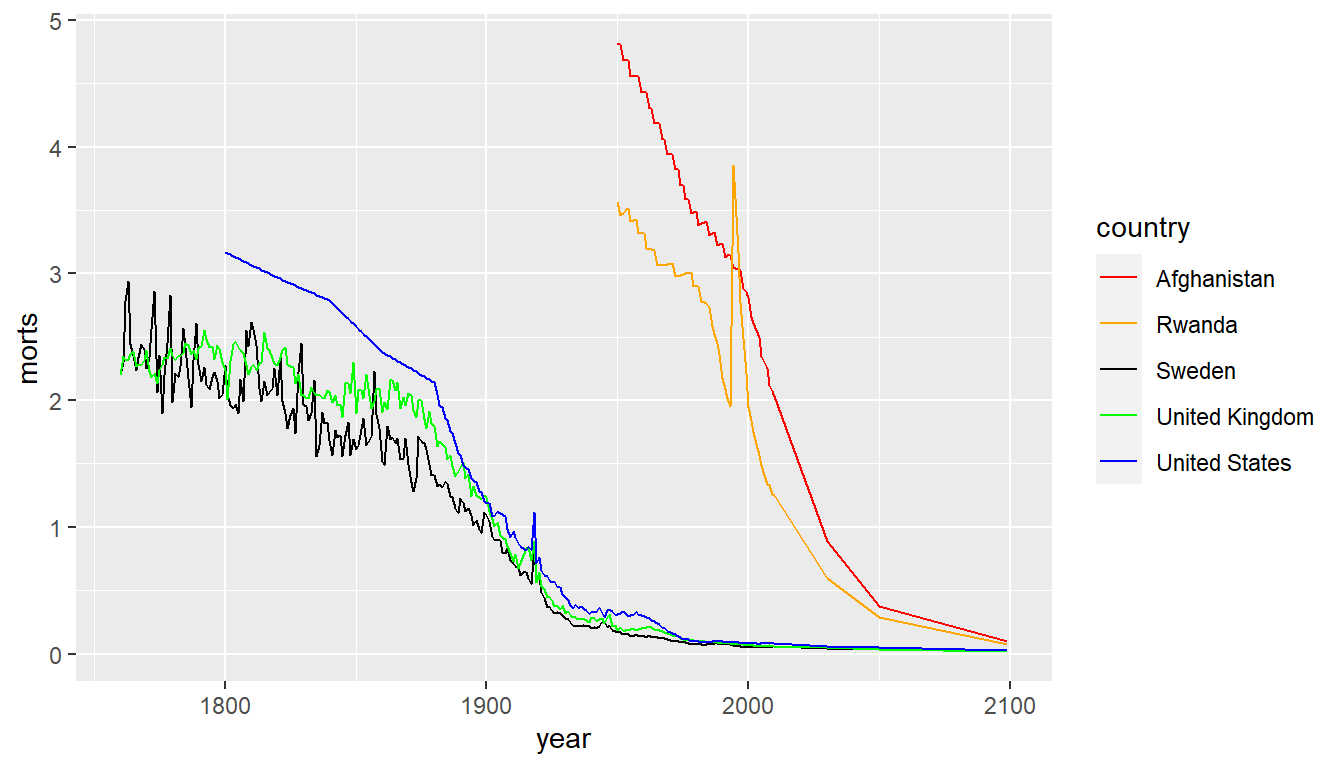## ggplot2: grouping - using colour

Let’s remove the legend using the `guide` command:

`g + geom_line() + guides(colour = FALSE)`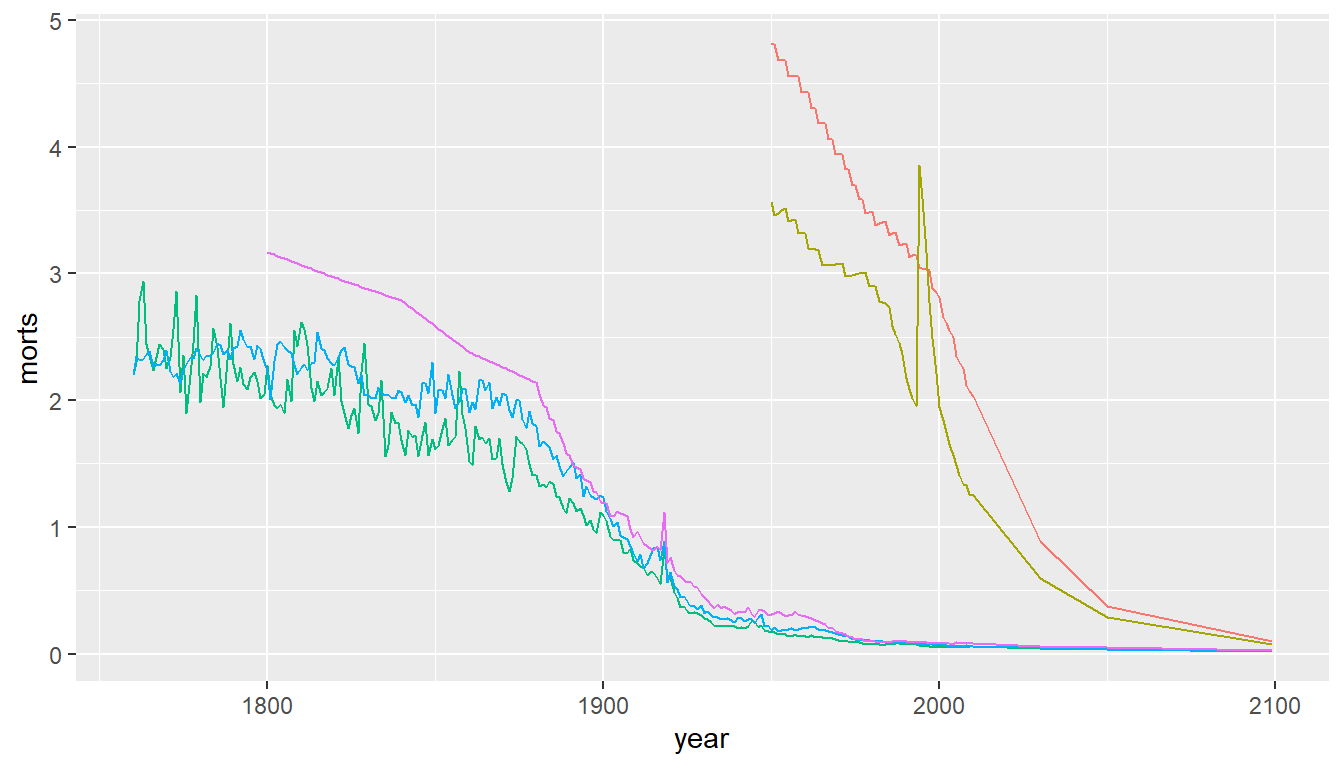## ggplot2: boxplot

`ggplot(long, aes(x = year, y = morts)) + geom_boxplot()`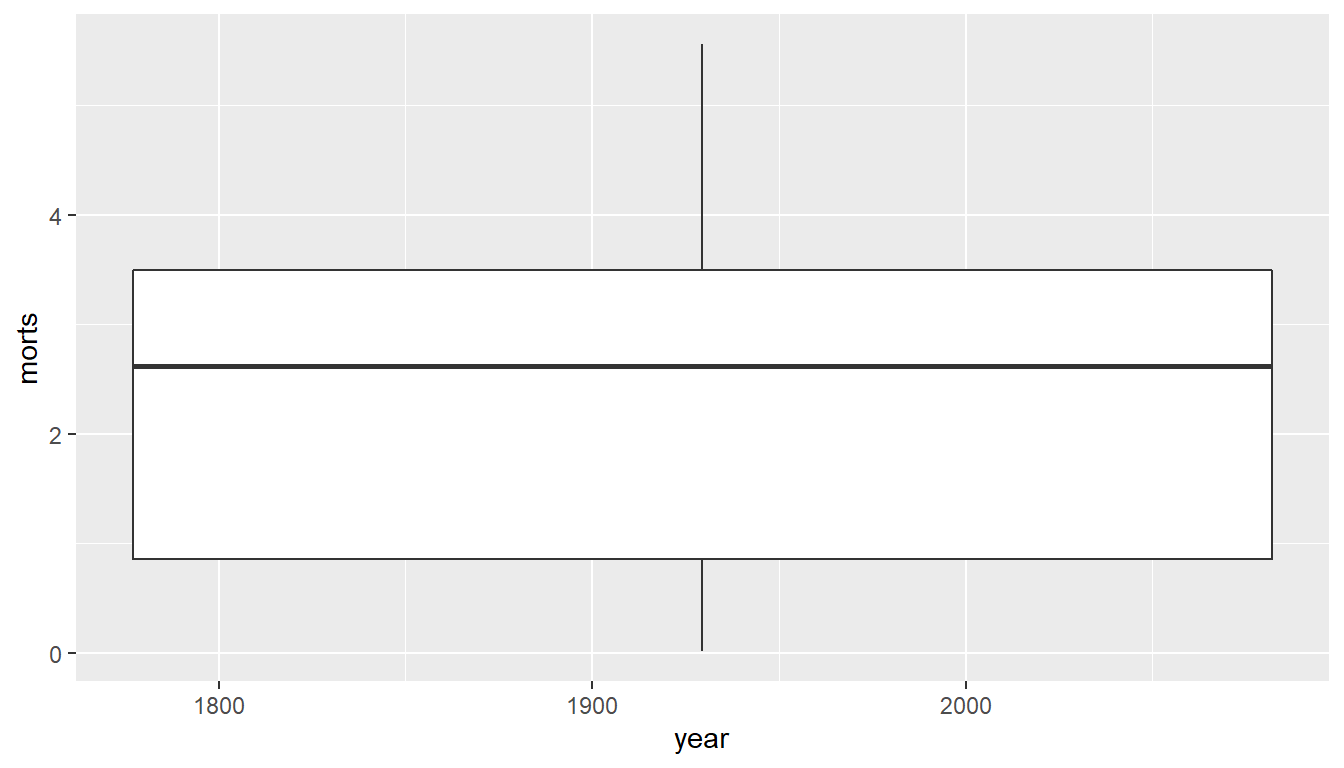## ggplot2: boxplot

For different plotting per year - must make it a factor - but x-axis is wrong!

`ggplot(long, aes(x = factor(year), y = morts)) + geom_boxplot()`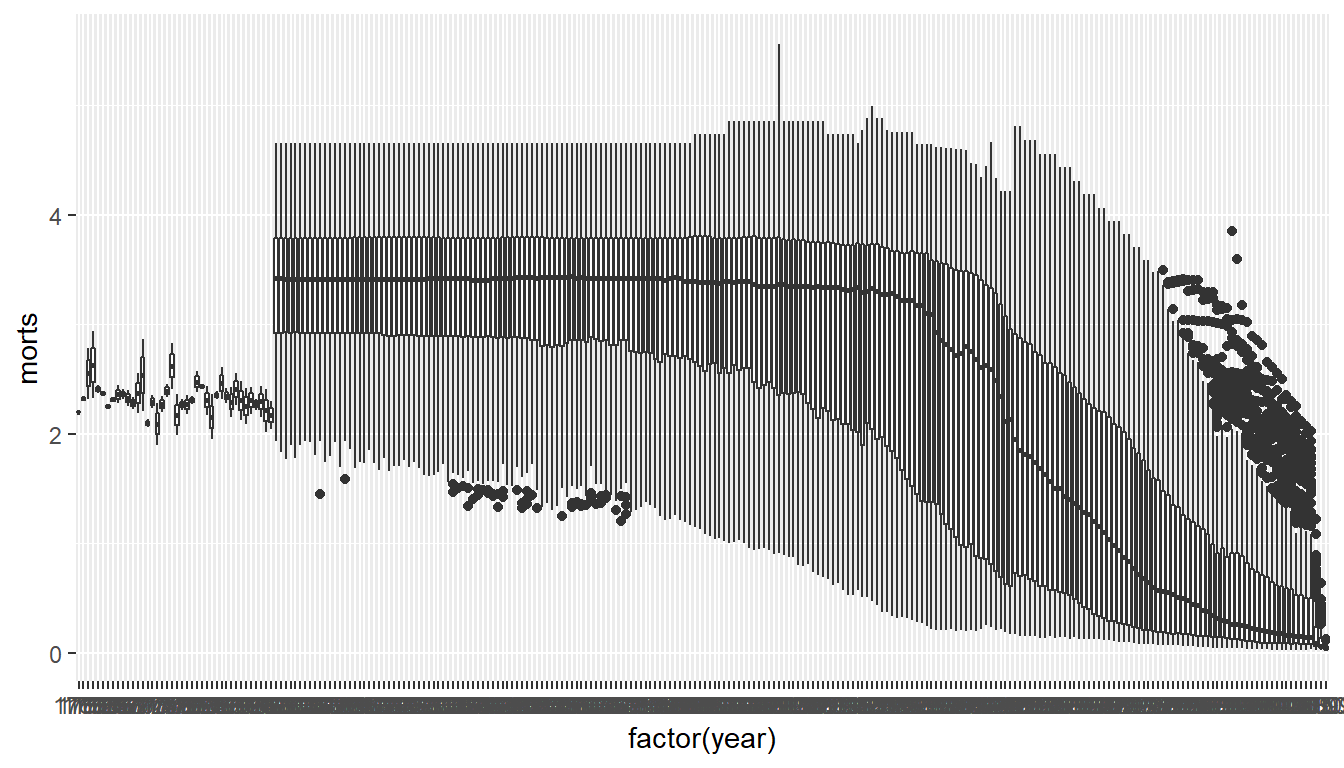## ggplot2: boxplot

`ggplot(long, aes(x = year, y = morts, group = year)) + geom_boxplot()`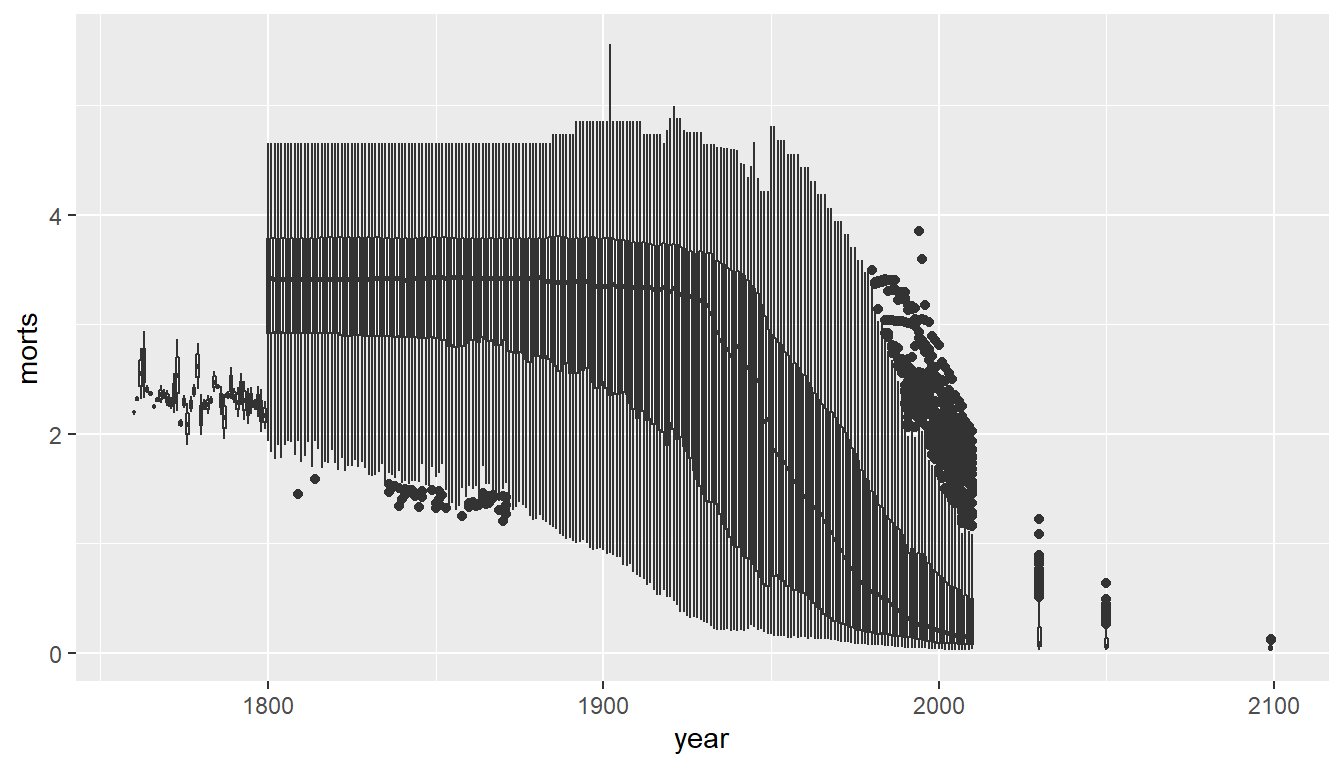## ggplot2: boxplot with points

• `geom_jitter` plots points “jittered” with noise so not overlapping
```sub_year = long %>% filter( year > 1995 & year <= 2000)
ggplot(sub_year, aes(x = factor(year), y = morts)) +
geom_boxplot(outlier.shape = NA) + # don't show outliers - will below
geom_jitter(height = 0)```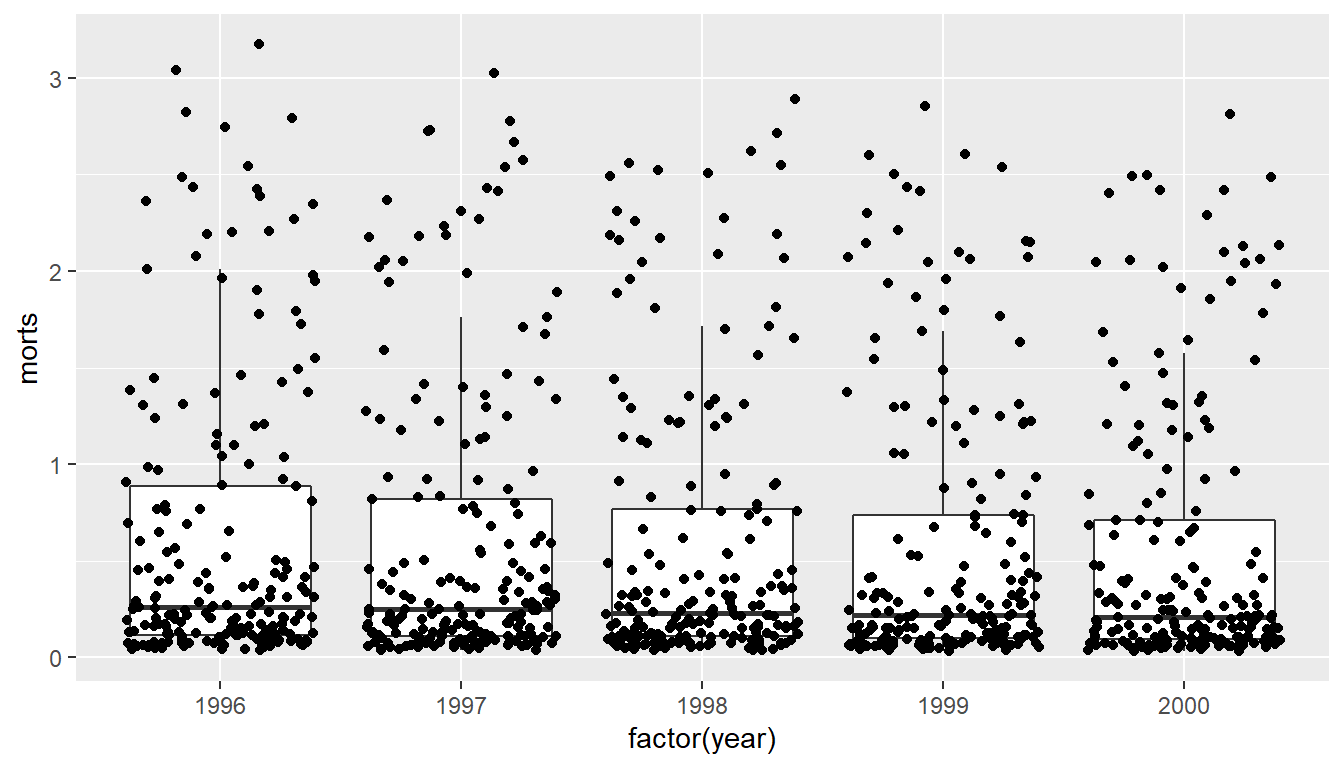## facets: plotting multiple panels

A `facet` will make a plot over variables, keeping axes the same (out can change that):

```sub %>% ggplot(aes(x = year, y = morts)) +
geom_line() +
facet_wrap(~ country)```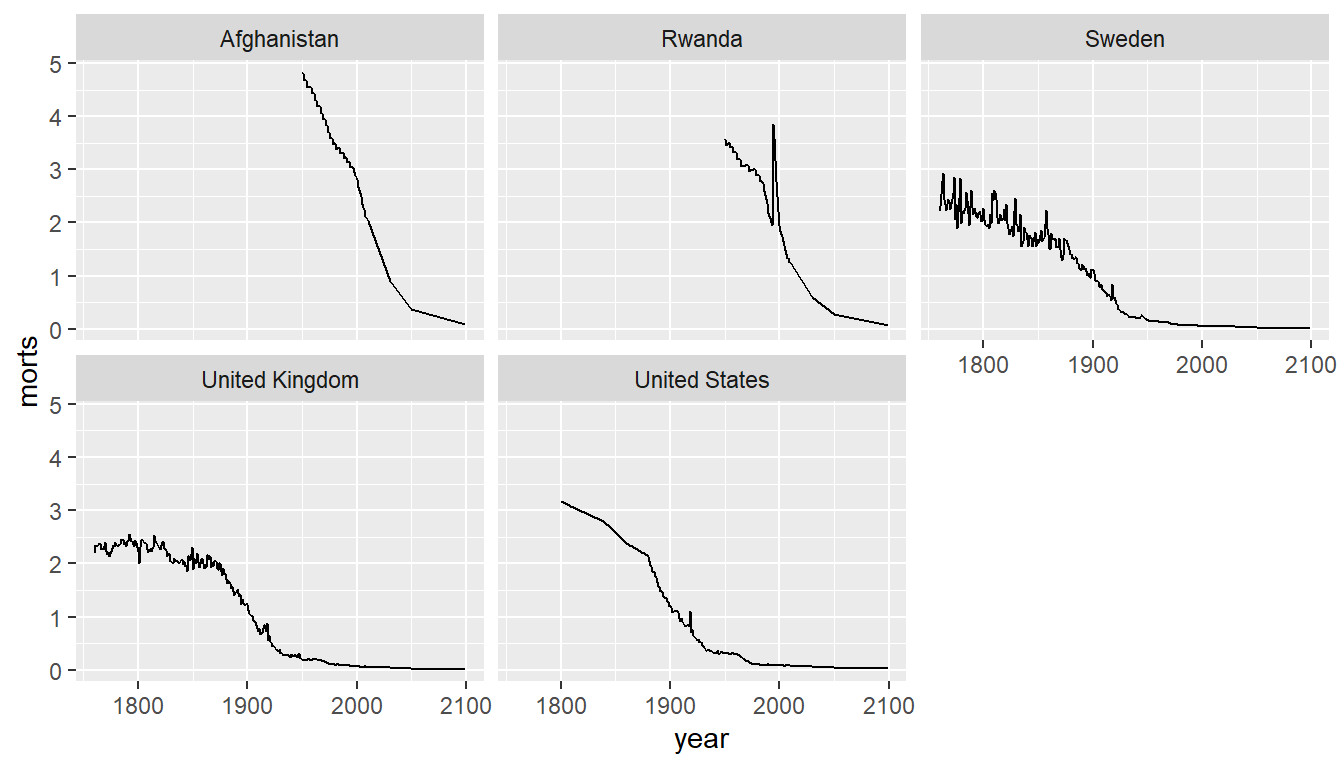## facets: plotting multiple panels

```sub %>% ggplot(aes(x = year, y = morts)) +
geom_line() +
facet_wrap(~ country, ncol = 1)```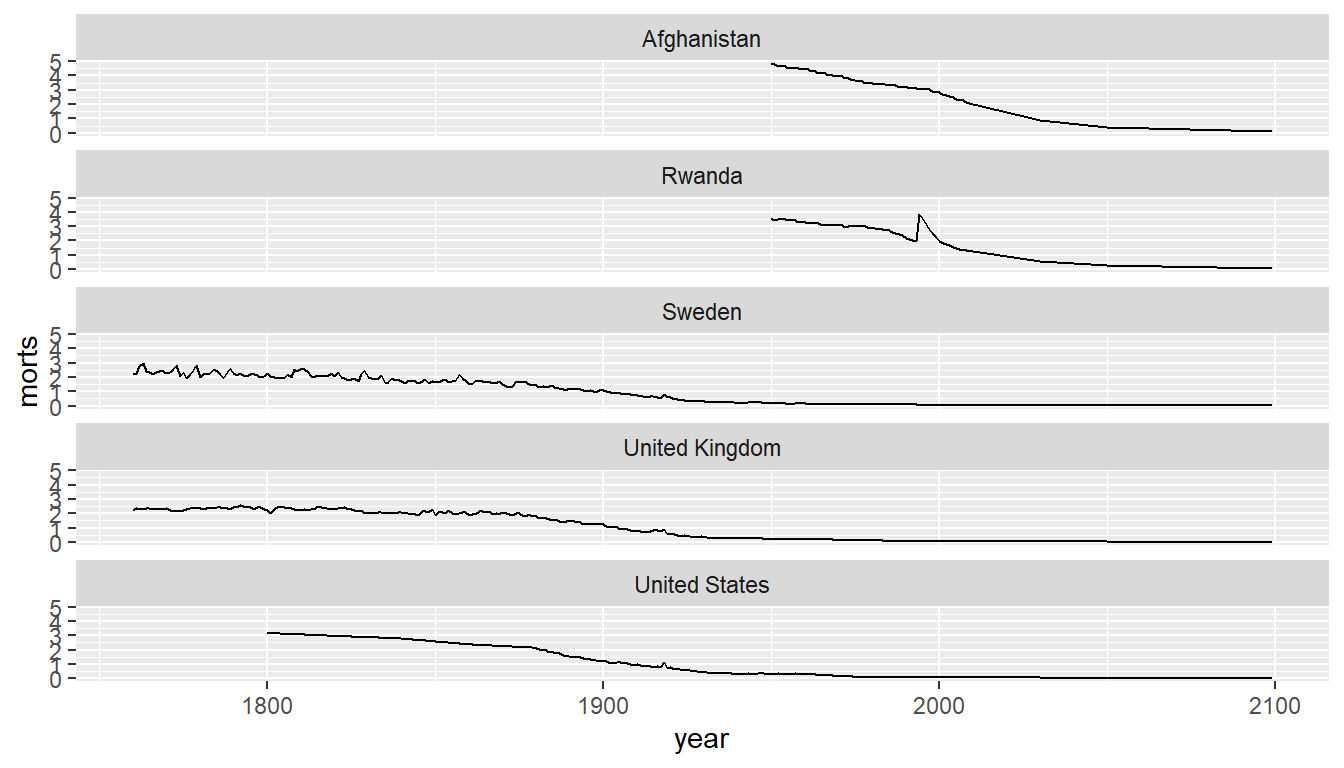## facets: plotting multiple panels

You can use `facets` in `qplot`

`qplot(x = year, y = morts, geom = "line", facets = ~ country, data = sub)`## facets: plotting multiple panels

You can also do multiple factors with `+` on the right hand side

```sub %>% ggplot(aes(x = year, y = morts)) +
geom_line() +
facet_wrap(~ country + x2 + ... )```

## Devices

By default, R displays plots in a separate panel. From there, you can export the plot to a variety of image file types, or copy it to the clipboard.

However, sometimes its very nice to save many plots made at one time to one pdf file, say, for flipping through. Or being more precise with the plot size in the saved file.

R has 5 additional graphics devices: `bmp()`, `jpeg()`, `png()`, `tiff()`, and `pdf()`

## Devices

The syntax is very similar for all of them:

```pdf("filename.pdf", width=8, height=8) # inches
plot() # plot 1
plot() # plot 2
# etc
dev.off()```

Basically, you are creating a pdf file, and telling R to write any subsequent plots to that file. Once you are done, you turn the device off. Note that failing to turn the device off will create a pdf file that is corrupt, that you cannot open.

## Saving the output:

```png("morts_over_time.png")
print(q)
dev.off()```
```png
2 ```
`file.exists("morts_over_time.png")`
` TRUE`

## Saving the output

There’s also a `ggsave` function that is useful for saving a single `ggplot` object.

## Labels and such

• `xlab`/`ylab` - functions to change the labels; `ggtitle` - change the title
```q = qplot(x = year, y = morts, colour = country, data = sub,
geom = "line") +
xlab("Year of Collection") + ylab("morts /100,000") +
ggtitle("Mortality of Children over the years", subtitle = "not great")
q```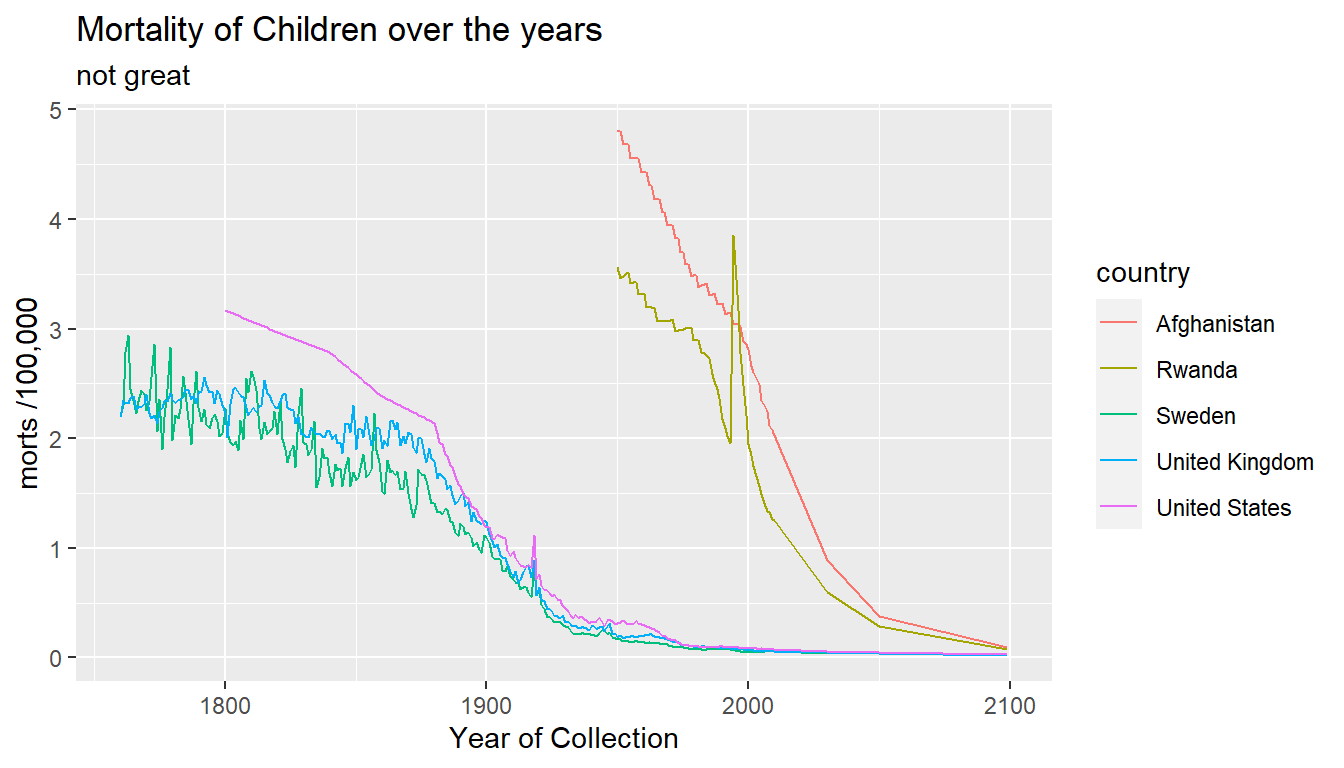## Themes

• see `?theme_bw` - for ggthemes - black and white
`q + theme_bw()`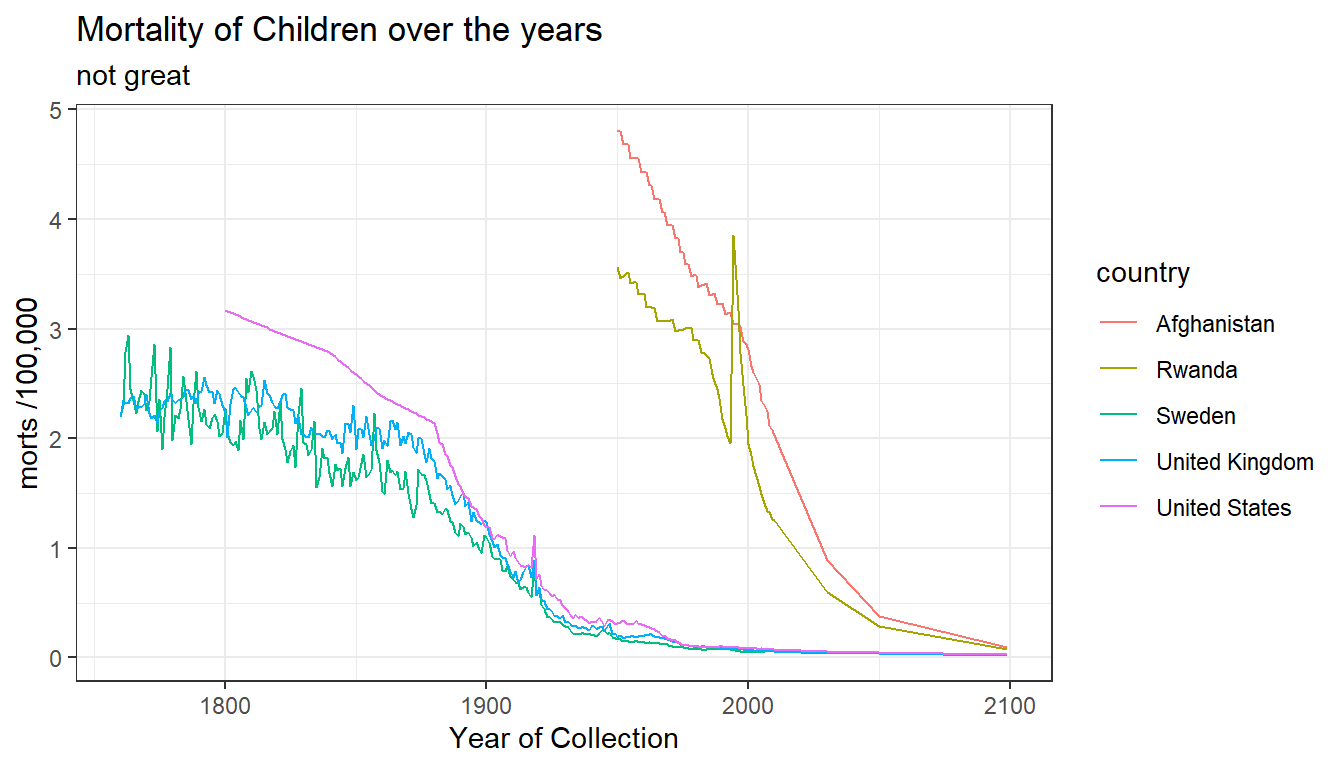## Themes: change plot parameters

• `theme` - global or specific elements/increase text size
`q + theme(text = element_text(size = 12), title = element_text(size = 20))`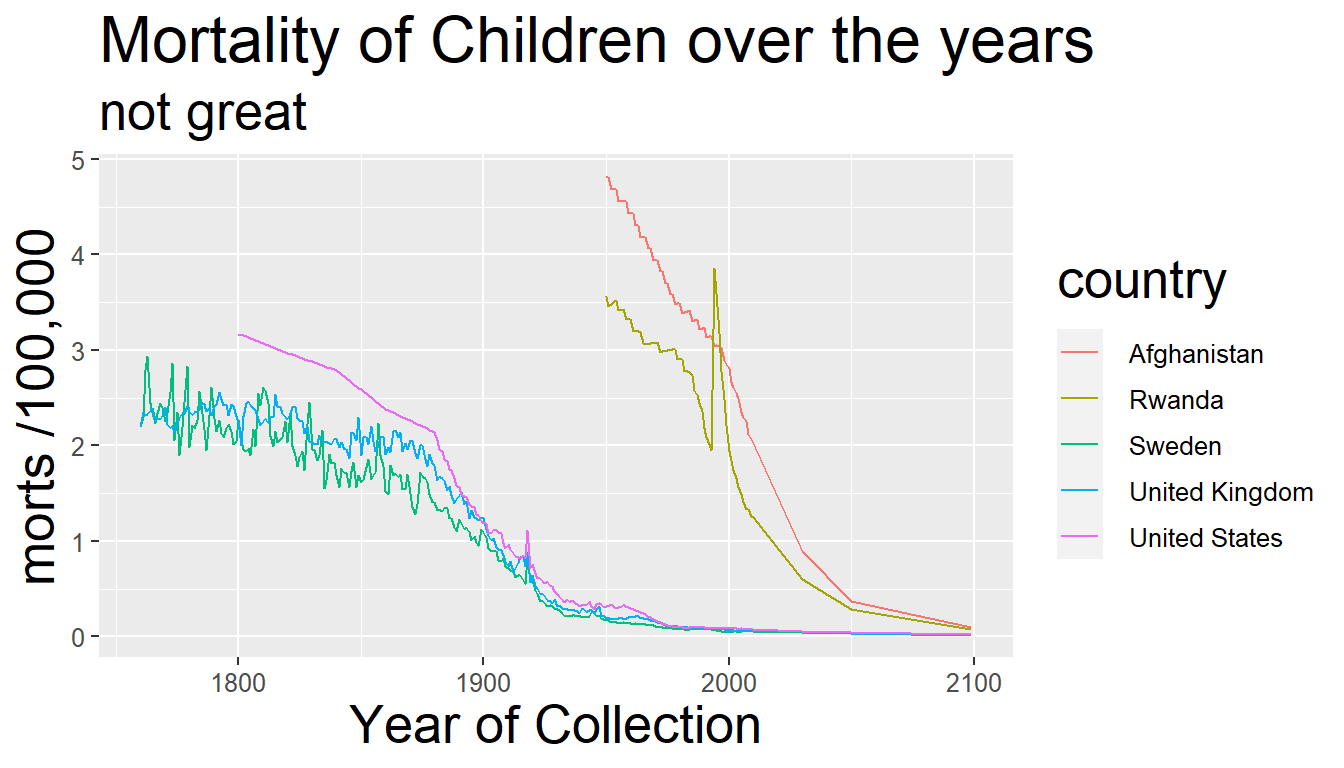## Themes

```q = q + theme(axis.text = element_text(size = 14),
title = element_text(size = 20),
axis.title = element_text(size = 16),
legend.position = c(0.9, 0.8)) +
guides(colour = guide_legend(title = "Country"))
q```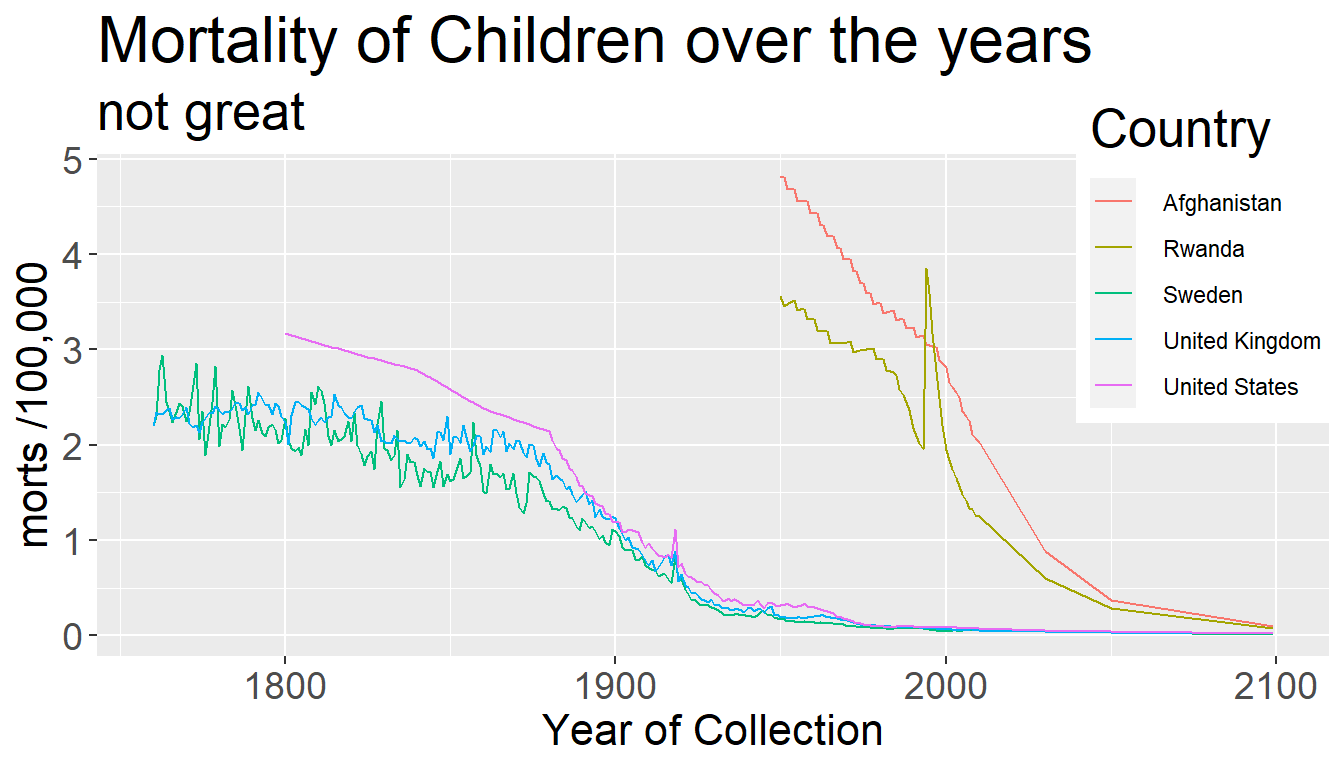## Code for a transparent legend

```transparent_legend =  theme(legend.background = element_rect(
fill = "transparent"),
legend.key = element_rect(fill = "transparent",
color = "transparent") )
q + transparent_legend```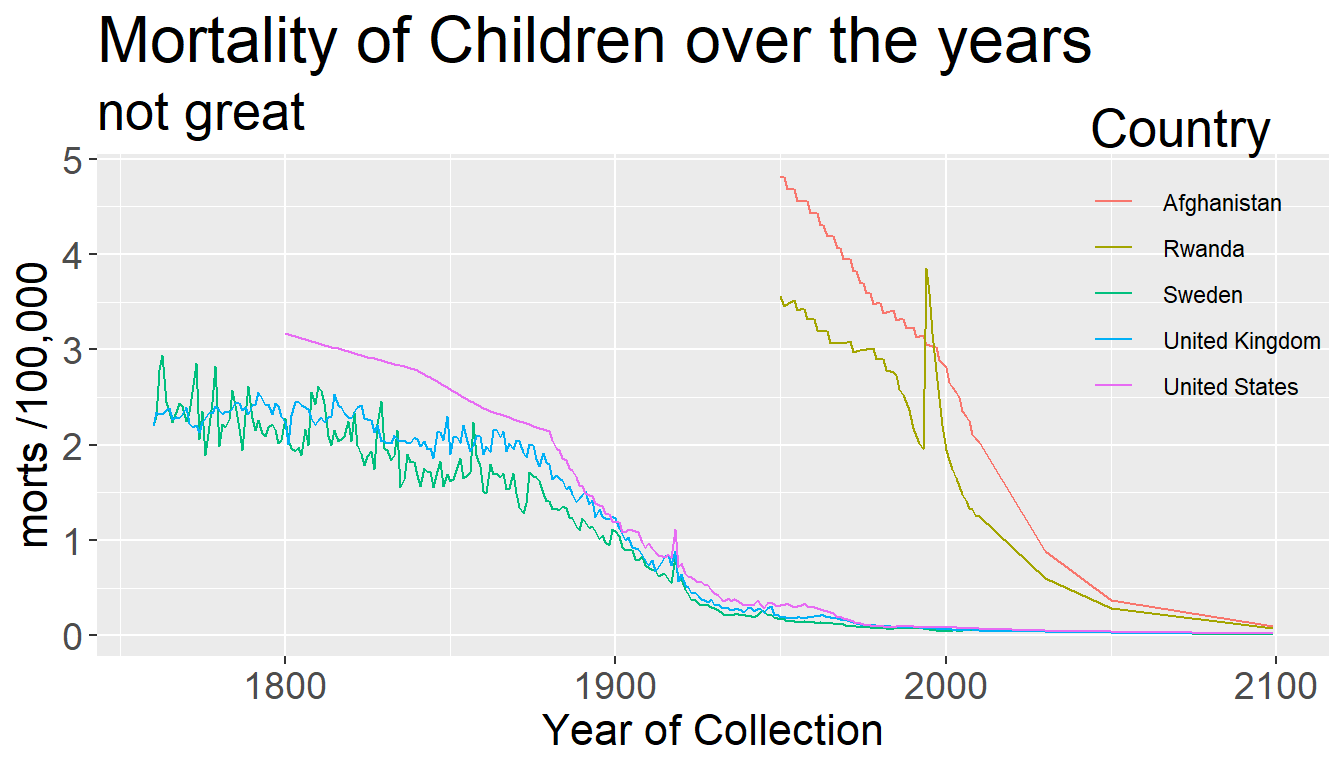## Histograms again: Changing bins

`qplot(x = morts, data = sub, bins = 200)`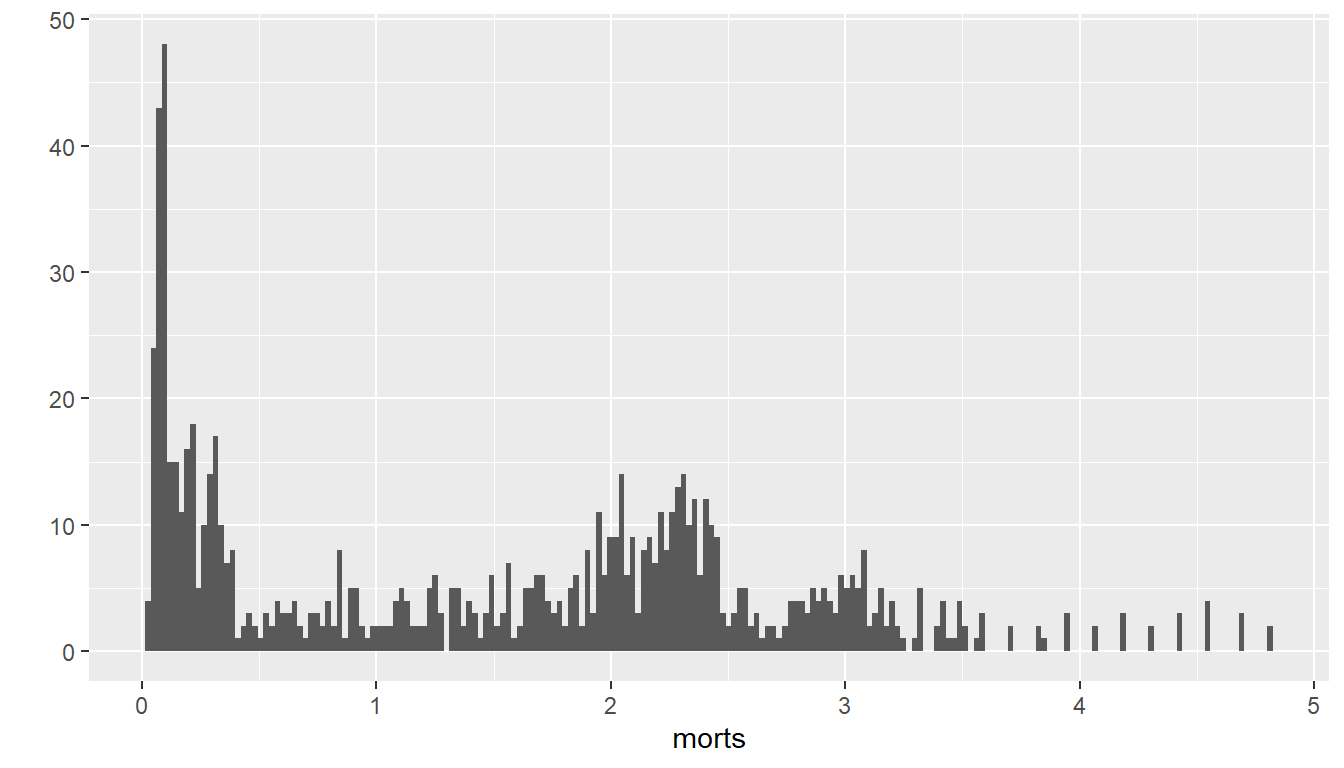## Multiple Histograms

```qplot(x = morts, fill = factor(country),
data = sub, geom = c("histogram"))```